96.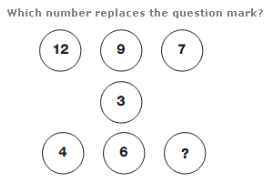A. 7 B. 4 C. 9 D. 3

Explanation: :

Starting with the numbers in the top row, and following straight lines through the centre of the diagram, subtract 3 from the number and put the result in the corresponding lower circle.

97.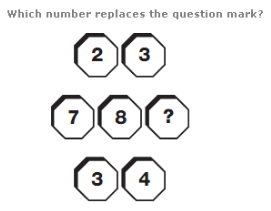A. 3 B. 5 C. 2 D. 4

Explanation: :

Reading the top and bottom lines as 2 digit numbers, multiply these together to give the 3 digit result, written in the middle.

98.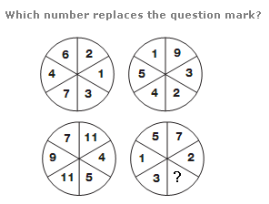A. 4 B. 1 C. 6 D. 7

Explanation: :

Numbers in the segments of the lower left circle equal the sum of the digits in corresponding segments of the upper circles.

Numbers in the lower right circle equal the difference between numbers in corresponding segments of the upper circles.

99.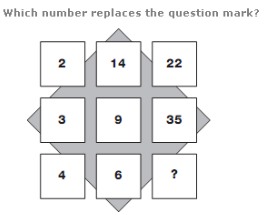A. 65 B. 78 C. 56 D. 44

Explanation: :

Starting at the top left and moving down, then to the right and up, in a snakes and ladders pattern, add the first two numbers together and subtract 1 to get the next in the sequence.

100.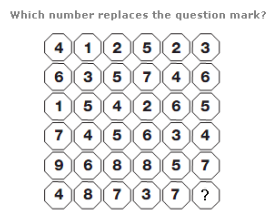A. 8 B. 3 C. 6 D. 1